# Lesson 5: Using LINEST for Property Appraisal

The value of a home (important to know for purposes of taxation or real estate sales) depends on a number of factors. Typically appraisals are done based by looking at recent sales of "similar" homes. Let's see how this can be done using Excel's LINEST tool.

We'll assume the that the value of a home depends only on:

• age (measured in years)
• number of bathrooms
• number of bedrooms
• heated area (measured in square feet), and
• "general condition" (a somewhat subjective measure for which a higher number is better)

# The Mathematical Model

We're going to assume that the value of a home depends linearly on the five criteria, at least in comparison to "comparable" homes (that is, recent sales with criterion values similar to the home whose value we'd like to estimate). That is, we assume: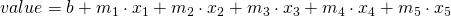where the parameters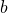and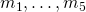are to be found and the variables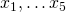are as recorded in this table: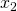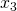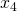age bathrooms bedrooms area general condition

This model is a linear function of five variables; LINEST will compute a "least-squares" solution to it whose analytic explanation is (just) a bit beyond us at the moment.

# The Computer Method

This is the same as Lesson 3, but now we have more independent variables (5 instead of 2), so LINEST needs more space. First let's enter the last seven comparable home sales into an Excel sheet:The output of LINEST will be the constant termand the five slopes. So we select a six-cell strip and enter "=LINEST(F2:F8,A2:E8)" to estimate the values in column F as a function of the data in columns in A through E.Then, remembering that LINEST is an array function, we evaluate using COMMAND-SHIFT-ENTER. We get a list of numbers in reverse order (Excel is weird sometimes), which I've labeled:So our linear model is (after rounding to the nearest penny):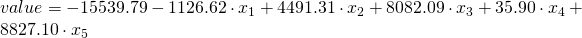Let's take a moment to interpret each of these numbers, just to make a bit of sense of them (and to understand why sometimes they don't make sense. The units are helpful here.

•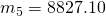The units are dollars-per-condition-point. This means that, for each additional "condition point" you get your home (say by repainting the exterior, or replacing the roof, or something) the value should increase by $8,827.10. This is must be done keeping all other variables equal. •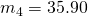The units are dollars-per-squre-foot. Each additional square foot you could add to your home would increase the value by$35.90. This is must be done keeping all other variables equal.
•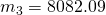The units are dollars-per-bedroom. Each additional bedroom (again, keeping all other variables equal, so you couldn't have added any additional square footage in the process) should add $8,082.09 to the value of the home. •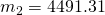The units are dollars-per-bathroom. Each additional bathroom (again, keeping all other variables equal, so you couldn't have added any additional square footage in the process) should add$4,491.31 to the value of the home.
•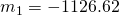The units are dollars-per-year. Your home is worth $1126.62 less than it was last year, assuming it hasn't deteriorated at all (that would affect its condition) and you haven't done anything to improve it. •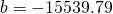The units are dollars. A brand-new home with zero bedrooms, zero bathrooms, zero square feet, and in absolutely terrible condition should have a value of$-15539.79. I guess this would mean an empty lot with a very small shack that was just built would actually be worth negative money. (To me this seems pretty unreasonable: a parcel of land is at least worth something, unless it's a SuperFund site or something.)

Always round only at the very end of a problem, when you're reporting your results. Why? Because rounding errors accumulate throughout a problem. Our intermediate results (the output of LINEST) are slopes, which means any errors we introduce by rounding will be multiplied by something. So it's best to work with as many decimal places as we can throughout the whole problem, and only round at the very last minute.

Now let's use our model to estimate the value of a 13-year-old home with 1800 square feet, 3 bedrooms, and 2.5 baths, and in excellent (9) condition. Actually let's write an Excel formula to do this for us:I'll let you sort out why I've used the formula I've used. The result is, after rounding, a value of $149,352.86. ### How good was the model? Now let's say that we put the home in question on the market, at$160,000 with the idea to negotiate down if needed. But a lovely family comes in, takes a look, and buys the house outright for the $160,000 asking price without asking. How should we describe the fact that our predicted sales price and the actual sales price were different? One way would be just to say: we underestimated by$10,647.14.

Now ten thousand dollars to me is a lot of money, but to Beyoncé it's not so much money. I'd very happy with my realtor in this situation; Queen Bee, wonderful though she is, might not even notice. So the number \$10,647.14 doesn't tell the whole story.

In a scientific lab class, where the goal is to learn to perfect your lab technique, you may see the formula "(measured - theoretical)/theoretical * 100" for percent error. This is because often in lab sciences there's a 'theoretical' value (the speed of light, or the acceleration due to gravity at sea level, or the freezing point of water) that is known and well-established, against which we're comparing our measurements (which we imagine might be wrong). But in this class, the "really real" thing is what actually happened, and it's our theoretical model that we're treating as potentially being wrong.

A standard way to report error that gets around this is to report percent error. That is, we report the error as a fraction of our original prediction: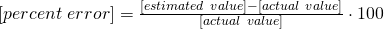In our case, this amounts to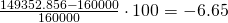We say the percent error is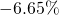.

# Takeaways/Deliverables

To say you've successfully completed this lesson, you should be able to do the following:

1. Use the LINEST command in Excel
2. Correctly interpret the output (parameters and correlation coefficient) of the LINEST command.
3. Use the output of LINEST to project or extrapolate a linear model from given data.
4. Compute percent error.

These skills are what you'll need to complete the WebAssign homework for this Lesson.